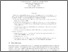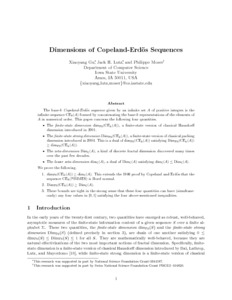# Dimensions of Copeland-Erdos Sequences

Gu, Xiaoyang and Lutz, Jack H. and Moser, Philippe (2007) Dimensions of Copeland-Erdos Sequences. Information and Computation, 205 (9). pp. 1317-1333. ISSN 0890-5401Preview
Download (195kB) | Preview

Share your researchmore...Add this article to your Mendeley library

## Abstract

The base-k Copeland-Erdös sequence given by an infinite set A of positive integers is the infinite sequence CEk(A) formed by concatenating the base-k representations of the elements of A in numerical order. This paper concerns the following four quantities. • The finite-state dimension dimFS(CEk(A)), a finite-state version of classical Hausdorff dimension introduced in 2001. • The finite-state strong dimension DimFS(CEk(A)), a finite-state version of classical packing dimension introduced in 2004. This is a dual of dimFS(CEk(A)) satisfying DimFS(CEk(A)) ≥ dimFS(CEk(A)). • The zeta-dimension Dimζ(A), a kind of discrete fractal dimension discovered many times over the past few decades. • The lower zeta-dimension dimζ(A), a dual of Dimζ(A) satisfying dimζ(A) ≤ Dimζ(A). We prove the following. 1. dimFS(CEk(A)) ≥ dimζ(A). This extends the 1946 proof by Copeland and Erdös that the sequence CEk(PRIMES) is Borel normal. 2. DimFS(CEk(A)) ≥ Dimζ(A). 3. These bounds are tight in the strong sense that these four quantities can have (simultane-ously) any four values in [0, 1] satisfying the four above-mentioned inequalities.

Item Type: Article This is the preprint version of the published article, which is available at DOI: 10.1016/j.ic.2006.01.006 Normality; Finite-state dimension; Copeland-Erdos Sequences; Faculty of Science and Engineering > Computer Science 8241 https://doi.org/10.1016/j.ic.2006.01.006 Philippe Moser 25 May 2017 15:48 Information and Computation Elsevier Yes

### Repository Staff Only(login required)Item control page

### Downloads

Downloads per month over past year